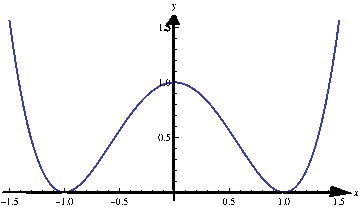# Maximum and Minimum of a Function

## Local maximum and minimum

Consider the function $$f(x)=x^4-2x^2+1$$. Without using too much theory we can express $$f$$ in the following way: $f(x)=\left(x^2-1\right)^2=(x-1)^2\cdot (x+1)^2.$ From this expression we see that the function is always non-negative and its minimum $$0$$ is attained at $$2$$ points: $$-1$$ and $$1$$. On the real line, the function does not have maximum, because as $$x$$ approaches infinity, the value of $$f$$ goes to infinity.

Let us now take a look at the graph of the function $$f$$:The graph shows that the minimum is $$0$$ at two points: $$-1$$ and $$1$$. However, the graph shows that the point $$x=0$$ is special: the function has a peak’’ at that point. It is not real maximum (because we saw that the function goes to $$+\infty$$). This point is called local maximum.’’ We are now going to define this term precisely.

Definition (Local maximum)

The function $$f$$ attains a local maximum at point $$a$$ if there is an interval of the form $$(p,q)$$ that contains $$a$$ (i.e. $$p< a< q$$) such that $$f(x)\leq f(a)$$ for all $$x\in (p,q)$$.

In an analogous way we define the local minimum.

Definition (Local minimum)

The function $$f$$ attains a local minimum at point $$a$$ if there is an interval of the form $$(p,q)$$ that contains $$a$$ (i.e. $$p< a< q$$) such that $$f(x)\geq f(a)$$ for all $$x\in (p,q)$$.

## Application of differential calculus in finding the local extrema

Theorem

If the function $$f$$ attains local minimum or local maximum at the point $$a\in\mathbb R$$, and if $$f$$ is differentiable at $$a$$, then $$f^{\prime}(a)=0$$.

Notice that the previous theorem makes sense: If $$f$$ attains maximum or minimum at $$a$$, the tangent has to be horizontal at $$a$$, hence the slope is $$0$$. The previous proof is just a formal way of writing this observation.

2005-2021 IMOmath.com | imomath"at"gmail.com | Math rendered by MathJax